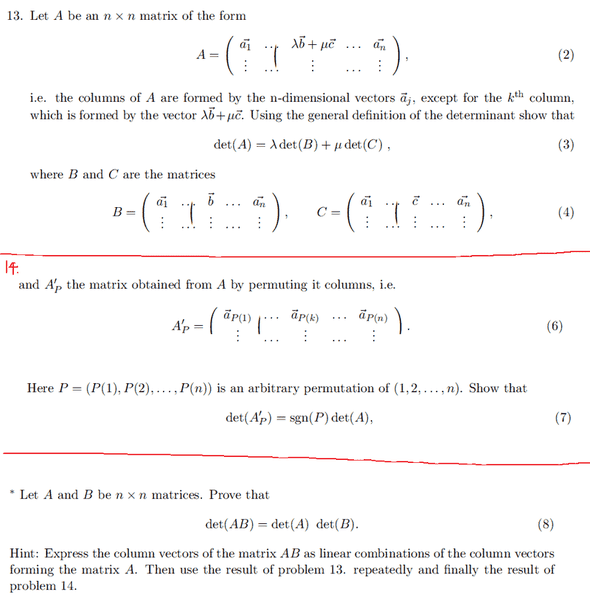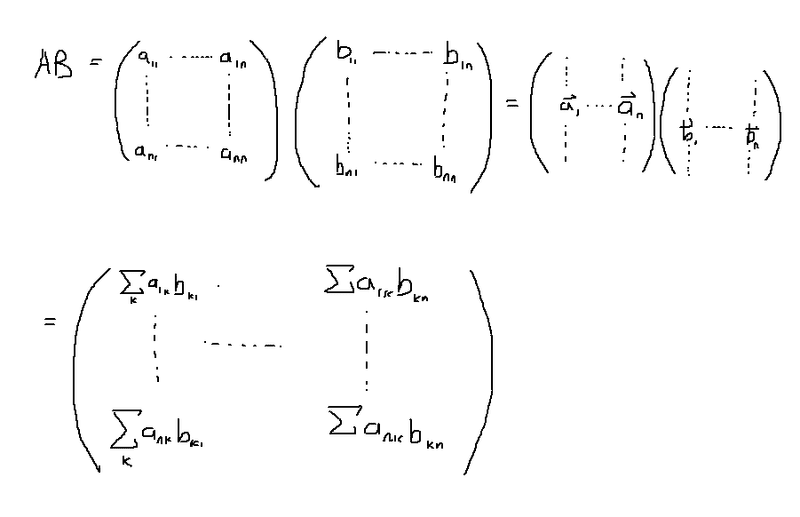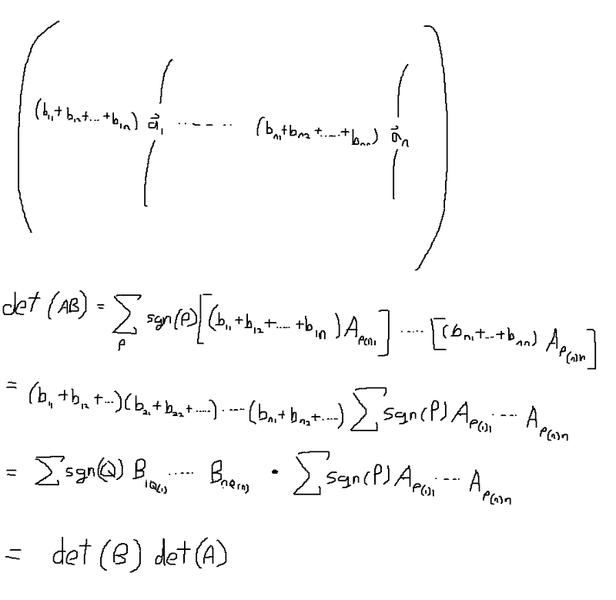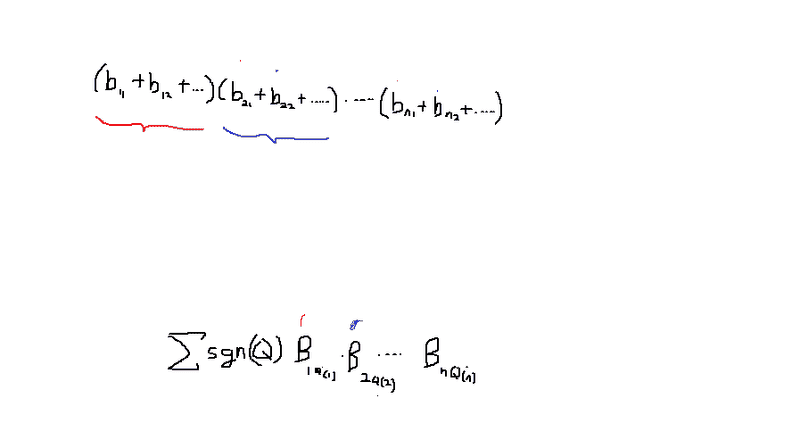# Proof on det(AB) = det(A)*det(B)

unscientific

## Homework Statement

Let A and B be nxn matrices, show that det(A)=det(A)det(B).## The Attempt at a SolutionI'm not sure how to express each column as a linear combination of vectors purely from A, or even from B. It's more of the dot product form: aT°b

## Answers and Replies

Homework Helper
I think you should tell us what your "general definition of the determinant" is. It's probably easiest to go directly from there.

unscientific
I think you should tell us what your "general definition of the determinant" is. It's probably easiest to go directly from there.

But i think we should first express the columns of matrix AB in terms of the columns of matrix A? (I'm not sure where B comes in) then somehow show that it is a linear combination, then make use of result 13. Then I think result 14 comes in when you get something that is just jumbled up, which simply gives det (AB) = sgn(P)*det(A)*det(B)

det(A) = Ʃ sgn(P) AP(1)1AP(2)2...

unscientific
bumpp

Staff Emeritus
Homework Helper
As you noted, the first column of AB is
$$\begin{pmatrix} \sum_{k=1}^n a_{1k}b_{k1} \\ \sum_{k=1}^n a_{2k}b_{k1} \\ \vdots \\ \sum_{k=1}^n a_{nk}b_{k1} \end{pmatrix} = \begin{pmatrix} a_{11}b_{11} + a_{12}b_{21} + a_{13}b_{31} + \cdots + a_{1n}b_{n1} \\ a_{21}b_{11} + a_{22}b_{21} + a_{23}b_{31} + \cdots + a_{2n}b_{n1} \\ \vdots \\ a_{n1}b_{11} + a_{n2}b_{21} + a_{n3}b_{31} + \cdots + a_{nn}b_{n1} \end{pmatrix}.$$ Express that in the form ##c_1\vec{a}_1 + c_2\vec{a}_2 + \cdots + c_n\vec{a}_n## where ##\vec{a}_k## is the kth column of A. How are the ##c_i##'s related to the elements of B?

unscientific
As you noted, the first column of AB is
$$\begin{pmatrix} \sum_{k=1}^n a_{1k}b_{k1} \\ \sum_{k=1}^n a_{2k}b_{k1} \\ \vdots \\ \sum_{k=1}^n a_{nk}b_{k1} \end{pmatrix} = \begin{pmatrix} a_{11}b_{11} + a_{12}b_{21} + a_{13}b_{31} + \cdots + a_{1n}b_{n1} \\ a_{21}b_{11} + a_{22}b_{21} + a_{23}b_{31} + \cdots + a_{2n}b_{n1} \\ \vdots \\ a_{n1}b_{11} + a_{n2}b_{21} + a_{n3}b_{31} + \cdots + a_{nn}b_{n1} \end{pmatrix}.$$ Express that in the form ##c_1\vec{a}_1 + c_2\vec{a}_2 + \cdots + c_n\vec{a}_n## where ##\vec{a}_k## is the kth column of A. How are the ##c_i##'s related to the elements of B?

So, the first column of AB = b11##\vec{a}_1## + b21##\vec{a}_2## + .... + bn1##\vec{a}_n##

kth-column = b1k##\vec{a}_1## + b2k##\vec{a}_2## + .... + bnk##\vec{a}_n##

I'm just not used to the idea of having "columns within a column", it sort of makes it more than nxn-dimensional.

Also, the earlier problem of

det(A) = λdet(B) + μdet(C)

only had one "special column" in matrix B and C that had a coefficient, while the rest were simply ##\vec{a}_1, \vec{a}_2, .... \vec{a}_n##

Last edited:
Staff Emeritus
Homework Helper
Good! So you've done what the first part of the hint suggested, expressing the columns of AB as linear combinations of the columns of A. Now just follow through with the rest of the hint.

unscientific
Good! So you've done what the first part of the hint suggested, expressing the columns of AB as linear combinations of the columns of A. Now just follow through with the rest of the hint.

Also, the earlier problem of

det(A) = λdet(B) + μdet(C)

only had one "special column" in matrix B and C that had a coefficient, while the rest were simply ##\vec{a}_1, \vec{a}_2, .... \vec{a}_n##

while in the new form, it's literally in every column.

unscientific
I think I got it:

We can simply rearrange the columns of matrix AB so as to separate them into the vectors ##\vec{a}_1, \vec{a}_2, \vec{a}_3 ....## and by result of problem 14, that's not going to change the absolute value of the determinant.

That would solve the dimensional crisis.Explanation why the product of b's = det(B)Consider the kth bracket, any permutation within that bracket is equivalent to a permutation of Q(k).